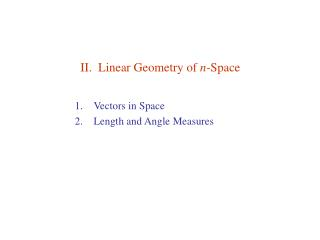Download PresentationII. Linear Geometry of n -Space

# II. Linear Geometry of n -Space - PowerPoint PPT PresentationDownload Presentation## II. Linear Geometry of n -Space

- - - - - - - - - - - - - - - - - - - - - - - - - - - E N D - - - - - - - - - - - - - - - - - - - - - - - - - - -
##### Presentation Transcript

1. II. Linear Geometry of n-Space • Vectors in Space • Length and Angle Measures

2. II.1. Vectors in Space See Apostol, Chap 1. Descartes: Points in plane ~ ( a1 , a2 ), points in space ~ ( a1 , a2 , a3 ). Cayley, Grassmann: Points in n-space ~ n-tuples ~ ( a1 , a2 , …, an)  Rn. Bound vector: a = ( a1 , a2 , …, an) = arrow from origin to point a. Vector algebra in Rn : For all a, b, c Rn and α R, 1. Vector equality: a = b ai= bi i = 1, …, n. 2. Vector addition: c = a + b ci= ai+ bi 3. Scalar Multiplication: a = αb  ai= α bi Closure: Rn is closed under all linear combinations of its elements:  Rn is a linear space Parallelogram rule:

3. Free vector: Object with a magnitude & a direction. 2 parallel arrows of the same length represent the same free vector.  bound vector of components bi ai. • Comment: • Free vectors can be defined only in a linear (flat) space. • In a (non-linear) curved space, parallelism must be defined explicitly. • ( Each point has its own linear vector space. ) • Riemannian geometry. • Differential geometry.

4. Rn is isomorphic to the space Mn1 of n1 column matrices. Definition: 2 spaces are isomorphic if there exists a bijection (1-1 onto mapping) between them that preserves the algebraic structures.    A real vector space is any space ( V, + ; R ) isomorphic to Rn .

5. Group Isomorphism Let { A, *} & {B, } be 2 groups. They are isomorphic if there exists a 1-1 onto mapping f s.t. f(a) = b where aA, bB Furthermore, f preserves the group operations, i.e., a1 * a2 = a3 f( a1 ) f( a2 ) = f( a3 ) Example I = [ 0, 2π) { I,  } is a group if we define  as { R,  } is a group if we define  as the matrix multiplication { I,  } & { R,  } are isomorphic with f : I → R by θ  R(θ)

6. Analytic Geometry Definition: Line A line spanned by a is the set of points L( 0; a ) = { ta | tR } [ L(0 ; a ) passes through 0 ] A line through point p & parallel to that spanned by a is the set of points L( p ; a ) = { p + ta | tR } Corollary: A line running through points p & q is the set of points L( p ; qp ) = { p + t (qp )| tR } Example: Line through points (1,2) & (3,1) in R2. Line through points (1,2) & parallel to (2,1).

7. Definition: Plane A plane spanned by a and b is the set of points P( 0 ; a, b ) = { sa + tb | s, tR } [ P(0 ; a, b ) passes through 0 ] A plane through point p and parallel to that spanned by a and b is the set of points P( p ; a, b ) = { p + sa + tb | s, tR } Corollary: A plane running through points p , q & r is the set of points P( p ; qp , rp ) = { p + s (qp ) + t (rp )| s, tR } Example: Plane through points (1, 0, 5), (2, 1, 3), & (2, 4, 0.5) in R3.

8. Example: Plane given by 2x + y + z = 4 in R3. Same as plane through points (2, 0, 0), (0, 4, 0), & (0, 0, 4) in R3.

9. Exercises 1.II.1. • 1. (a) Describe the plane through (1,1,5,1), (2, 2, 2,0), and (3, 1, 0,4). • (b) Is the origin in that plane? • A person traveling eastward at a rate of 3 miles per hour finds that the wind appears to blow directly from the north. On doubling his speed it appears to come from the north east. What was the wind’s velocity? • Intersect these planes:

10. II.2. Length and Angle Measures Definition 2.1: Euclidean norm (Length) The Euclidean norm || v || of a vector v Rn is Law of Cosines: Proof: Pythagorean theorem:

11. Example: Angle between 2 vectors. Law of Cosines:

12. Definition 2.3: Dot/Inner/Scalar Product  Law of Cosines: ( Definition 2.7 ) Exercise: Show that

13. Theorem 2.5: Triangle Inequality The sum of 2 edges of a triangle is greater than or equal to its 3rd edge. Shortest path between 2 points is a straight line. Geodesics in a Euclidean space are a straight lines. Proof: Since all norms are positive, the inequality is equivalent to or ( Cauchy-Schwarz Inequality ; to be proved ) Corollary: Setting v → v gives → →

14. Theorem 2.6: Cauchy-Schwarz Inequality Proof: Let where  a, b Let & → Conclusion: ||w||  0   Definition: Vectors u, v are orthogonal if u · v = 0.

15. Exercises 1.II.2.